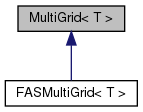Chombo + EB  3.2
MultiGrid< T > Class Template Reference

#include <MultiGrid.H>

Inheritance diagram for MultiGrid< T >:[legend]

Public Member Functions

MultiGrid ()

virtual ~MultiGrid ()

virtual void define (MGLevelOpFactory< T > &a_factory, LinearSolver< T > *a_bottomSolver, const ProblemDomain &a_domain, int a_maxDepth=-1, MGLevelOp< T > *a_finestLevelOp=NULL)
Function to define a MultiGrid object. More...

virtual void solve (T &a_phi, const T &a_rhs, Real a_tolerance, int a_maxIterations, int verbosity=0)

virtual void oneCycle (T &a_e, const T &a_res)
Execute ONE v-cycle of multigrid. More...

void init (const T &a_correction, const T &a_residual)

void cycle (int a_depth, T &a_correction, const T &a_residual)

void clear ()

void setBottomSolver (LinearSolver< T > *a_bottomSolver)

Vector< MGLevelOp< T > * > getAllOperators ()

Public Attributes

int m_depth

int m_defaultDepth

int m_pre

int m_post

int m_bottom

int m_cycle

int m_numMG

bool m_homogeneous

LinearSolver< T > * m_bottomSolver

ProblemDomain m_topLevelDomain

Protected Attributes

bool m_defined

int m_bottomCells

Vector< MGLevelOp< T > * > m_op

Vector< T * > m_residual

Vector< T * > m_correction

std::vector< bool > m_ownOp

Private Member Functions

MultiGrid (const MultiGrid< T > &a_opin)

void operator= (const MultiGrid< T > &a_opin)

Detailed Description

template<class T> class MultiGrid< T >

Class to execute geometric multigrid. This class is not meant to be particularly user-friendly and a good option for people who want something a tad less raw is to use AMRMultiGrid instead.

Constructor & Destructor Documentation

template<class T >
 MultiGrid< T >::MultiGrid ( )
template<class T >
 MultiGrid< T >::~MultiGrid ( )
virtual
template<class T>
 MultiGrid< T >::MultiGrid ( const MultiGrid< T > & a_opin )
inlineprivate

References MayDay::Error().

Member Function Documentation

template<class T >
 void MultiGrid< T >::define ( MGLevelOpFactory< T > & a_factory, LinearSolver< T > * a_bottomSolver, const ProblemDomain & a_domain, int a_maxDepth = -1, MGLevelOp< T > * a_finestLevelOp = NULL )
virtual

Function to define a MultiGrid object.

a_factory is the factory for generating operators. a_bottomSolver is called at the bottom of v-cycle. a_domain is the problem domain at the top of the vcycle. maxDepth defines the location of the bottom of the v-cycle. The vycle will terminate (hit bottom) when the factory returns NULL for a paticular depth if maxdepth = -1. Otherwise the vycle terminates at maxdepth.

Reimplemented in FASMultiGrid< T >.

Referenced by FASMultiGrid< T >::define().

template<class T >
 void MultiGrid< T >::solve ( T & a_phi, const T & a_rhs, Real a_tolerance, int a_maxIterations, int verbosity = 0 )
virtual

solve L(a_phi) = a_rhs. Tolerance is how much you want the norm of the error reduced. verbosity is how chatty you want the function to be. maxIterations is the maximum number of v-cycles. This does the whole residual correction switcharoo and calls oneCycle up to maxIterations times, evaluating the residual as it goes.

Reimplemented in FASMultiGrid< T >.

References CH_TIME, Max(), and pout().

template<class T >
 void MultiGrid< T >::oneCycle ( T & a_e, const T & a_res )
virtual

Execute ONE v-cycle of multigrid.

If you want the solution to converge, you need to iterate this. See solve() or AMRMultiGrid::solve for a more automatic solve() function. This operates residual-correction form of solution so all boundary conditions are assumed to be homogeneous. L(a_e) = a_res

Reimplemented in FASMultiGrid< T >.

References CH_TIME.

template<class T >
 void MultiGrid< T >::init ( const T & a_correction, const T & a_residual )

References CH_TIME.

template<class T >
 void MultiGrid< T >::cycle ( int a_depth, T & a_correction, const T & a_residual )

References CH_TIME.

template<class T >
 void MultiGrid< T >::clear ( )
template<class T >
 void MultiGrid< T >::setBottomSolver ( LinearSolver< T > * a_bottomSolver )
template<class T >
 Vector< MGLevelOp< T > * > MultiGrid< T >::getAllOperators ( )

for changing coefficients — not for the faint of heart.

template<class T>
 void MultiGrid< T >::operator= ( const MultiGrid< T > & a_opin )
inlineprivate

References MayDay::Error().

Member Data Documentation

template<class T>
 int MultiGrid< T >::m_depth

Public solver parameters. m_pre and m_post are the ones that usually get set and are the number of relaxations performed before and after multigrid recursion. See AMRMultiGrid for a more user-friendly interface.

template<class T>
 int MultiGrid< T >::m_defaultDepth
template<class T>
 int MultiGrid< T >::m_pre
template<class T>
 int MultiGrid< T >::m_post
template<class T>
 int MultiGrid< T >::m_bottom
template<class T>
 int MultiGrid< T >::m_cycle
template<class T>
 int MultiGrid< T >::m_numMG
template<class T>
 bool MultiGrid< T >::m_homogeneous
template<class T>
 LinearSolver* MultiGrid< T >::m_bottomSolver
template<class T>
 ProblemDomain MultiGrid< T >::m_topLevelDomain
template<class T>
 bool MultiGrid< T >::m_defined
protected
template<class T>
 int MultiGrid< T >::m_bottomCells
protected
template<class T>
 Vector*> MultiGrid< T >::m_op
protected
template<class T>
 Vector< T* > MultiGrid< T >::m_residual
protected
template<class T>
 Vector< T* > MultiGrid< T >::m_correction
protected
template<class T>
 std::vector MultiGrid< T >::m_ownOp
protected

The documentation for this class was generated from the following file: